# Category - Excel Tutorials

## Splitting Names in Excel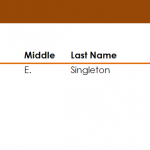Learn the process for splitting names in Excel spreadsheets. When importing information into Excel, name data usually ends up all in one column, making it …

## Get Sum in Excel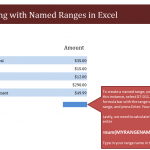Learn how to get sum in Excel spreadsheets from a group of numbers using simple formulas. When working with named ranges in Excel, you’ll be …

## Put Dashes in SSN in Excel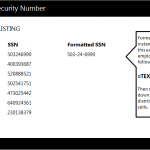Learn to put dashes in SSN in Excel spreadsheets with a simple formula. Follow our guide to learn how to input dashes in the right …

## Get Biggest Number in Excel Range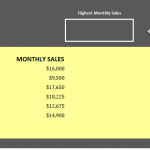Learn how to find the biggest number in Excel worksheets. Excel has a formula that allows a user to select a group of cells and …

## Find Smallest Number in Excel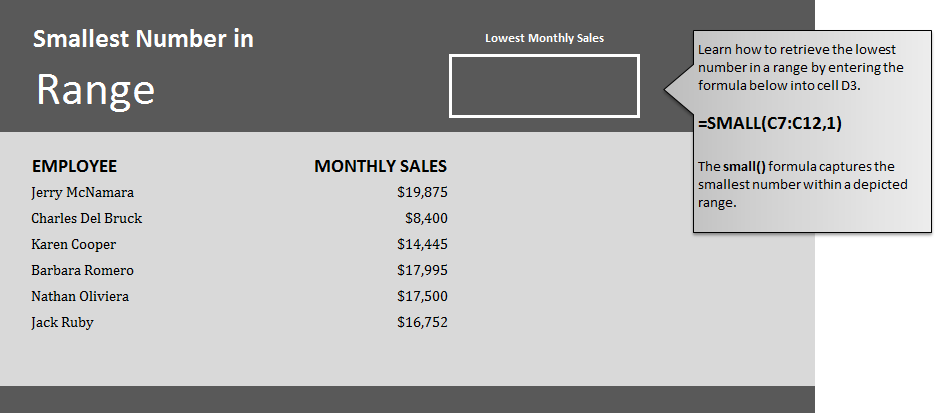This formula is used to find smallest number in Excel spreadsheets. Learn how to find the smallest number in a range within an Excel worksheet. …

## Find First Name in Excel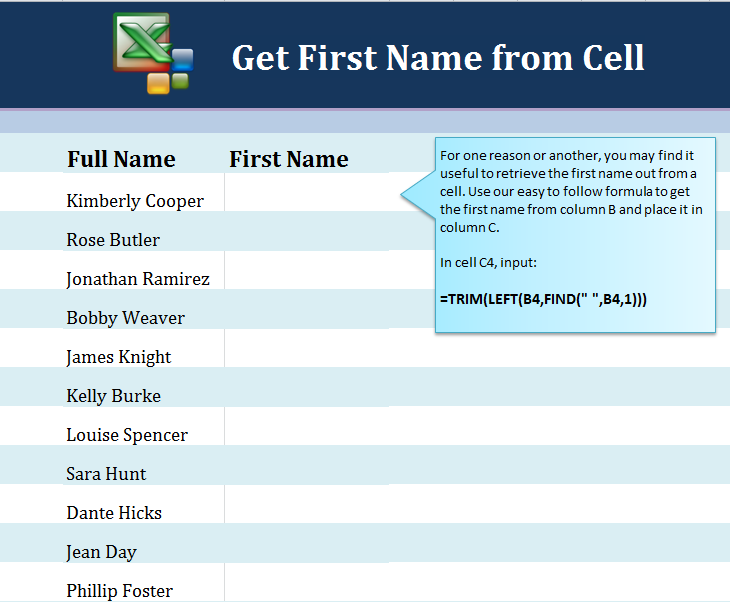Learn how to find first name in Excel spreadsheets with full names lists. If you have imported data from another source and inserted it into …

## Calculate Days Left in the Year in ExcelLearn how to calculate days left in the year in Excel spreadsheets to create your own countdown. Excel has stackable formulas you can use to …

## Count Cells with Text in Excel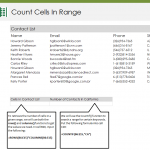Follow our guide and learn how to count cells with text in Excel spreadsheets. Excel has a formula that you can enter that will search …

## Retrieve Initials from Name in ExcelLearn how to make initials in Excel spreadsheets from someone’s full name. You can use a formula to retrieve initials from first, middle, and last …

## Eliminate Decimals in Excel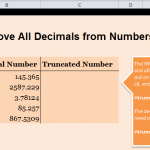Learn how to eliminate decimals in Excel spreadsheets to turn complicated numbers into normal whole numbers. Our guide will show you the simple formula to …

Page 2 of 5